# Excel Formulas: Make your job easy with the most useful excel formulas 2023.

Hello Friends, In this blog post(Make your job easy with the most useful excel formulas 2023), I am going to explain the ten most useful excel formulas. which can make your life easier if you are associated with excellent work in your professional life.

Within this blog we will explore how to How do you calculate in Excel?… How do you write formulas in Excel?… What are the most used formulas in Excel?|Make your job easy with the most useful excel formulas in 2023|

Most of us use this excel software for storing data or information regarding our personal or professional work.|Make your job easy with the most useful excel formulas 2023|

But very few of us know the real magic of this software or application like Excel and how to use excel formulas.|Make your job easy with the most useful excel formulas 2023|

### Are you really using this excel formula efficiently….? / How do you write formulas in Excel..?

If yes! then fine else you can learn a few basic but most useful formulas over here.

This tool is very powerful in terms of storing data, making automatic calculations over data, advanced searching of data, and much more…..

these excel formulas can really make your work very quick and easy whether you are using it at home or at your workplace.

this could be a great help in your job, and you can also grab a job by being professional in this.

How to use this formula and where is to write this formula…….?

### Here I am going to discuss Ten basic excel formulas that are most excessively used during work / What are the most used formulas in Excel?

1. SUM

Using excel formulas we can easily add up two and more columns even having complex numbers within a second.

If you want to sum the numbers of two-column say A and B and show the total sum in column C then you need to use the formula given below.

Formula: =SUM(col1, col2,…..) or   =SUM(A1,B1,……)

It is really simple to use this SUM formula.

Write the formula for the first cell and drag out the selection up to the full series of numbers:

Suppose you have numbers in two columns and you want to add them and show the result in the third column.

For doing this just write the formula in the first cell and then drag out the selection for the rest of the column.

For example, if you have a number in columns A and B and you want to show the sum in the third column C then you have to write

the formula in the C1 cell and then drag out the selection for the rest of the column, you will get the sum for the full column length of A and B.

See the image below: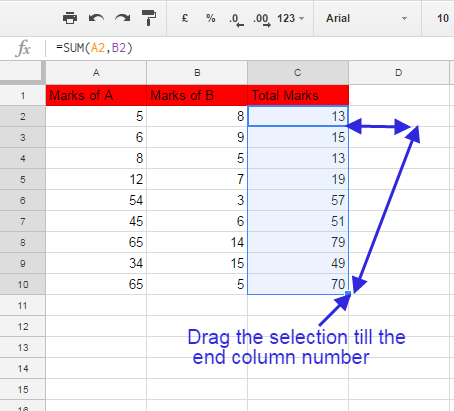2. TRIM

Formula: =TRIM(Column containing string)  OR  =TRIM(A1)

This formula is used for removing the extra spaces before and after the statement in the cell.

This is a very helpful formula while extracting the values from the database without introducing extra space.

See the image below.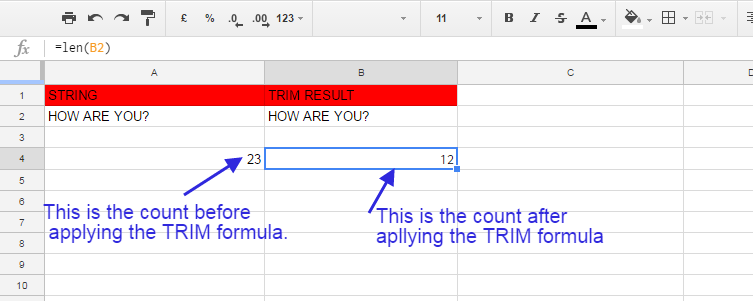3. LEN

Formula: =LEN(A1)  OR  =LEN(Column name)

This formula is used for counting the number of characters or digits in a cell.

See the image below: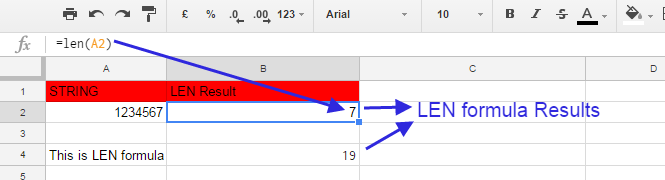4. COUNT

Formula: =COUNT(A1: A10)   OR  =COUNT(Range)

This formula counts all the cells in a range that have a number in the cells. The cells having characters are not included in this count.

Empty cells are not counted.

See the image below.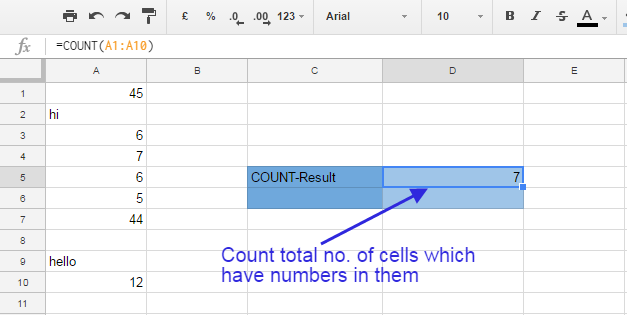5. COUNT-A

Formula: =COUNTA(A1:A10)     OR   =COUNT(RANGE)

This formula is used for counting all the cells containing the number and character both.

It does not count the empty cells.

See the image below: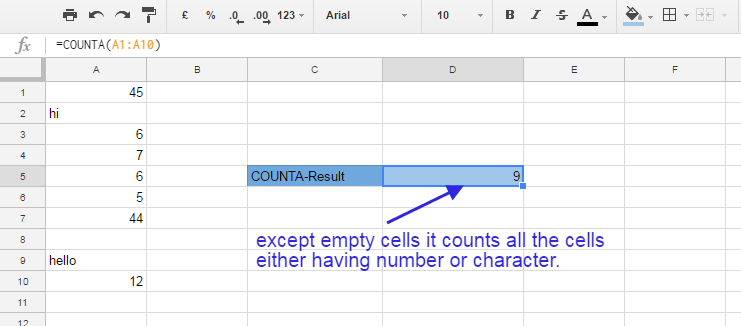6. RIGHT, LEFT, AND MID

RIGHT
Formula: = RIGHT(Text or cell reference, Number of characters)  OR   =RIGHT(A9,8)

See the image below: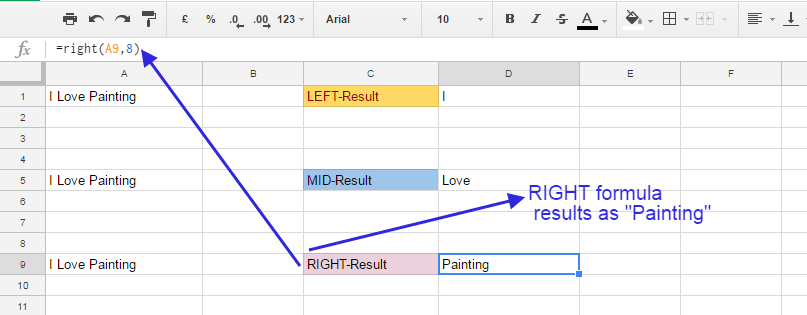MID

Formula: =MID(Text, start number, No. of characters)  OR  =MID(A5,3,4)

See the image below: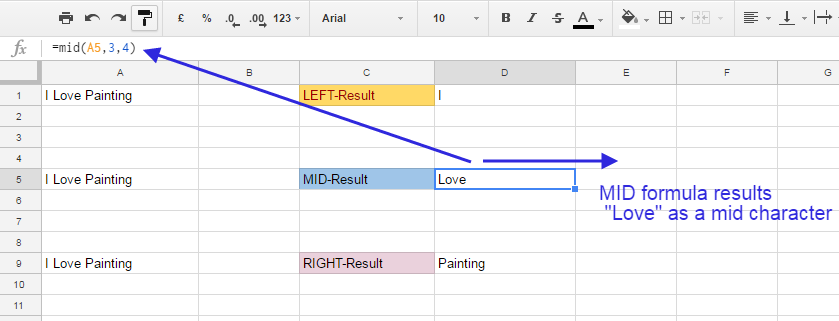LEFT

Formula: = LEFT(Text or cell reference or A1, Number of characters)  OR  =LEFT(A1,1)

See the image below: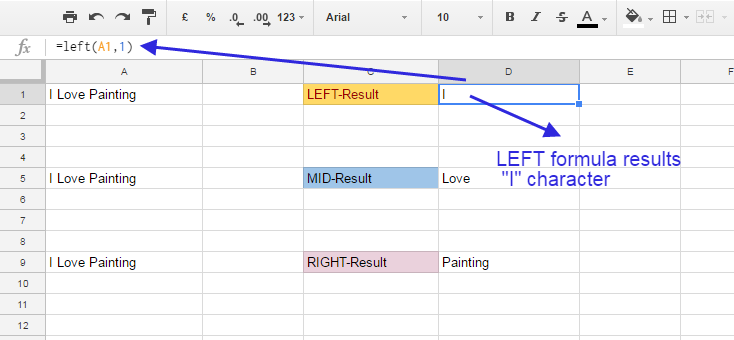7. VLOOKUP
Excel formulas VLOOKUP is a very useful formula in excel that is used to identify one or multiple values on the basis of simple and complex conditions.

Formula: =VLOOKUP(lookup_value, table_array, col_index_num, range_lookup)

OR   =VLOOKUP(E2,  \$A\$1:\$B\$7, 2, False)

Lookup_Value: It is a field for which we need to find a lookup_value.

Lookup_Array: It is the complete table or all possible values that need to have by looking up the desired values.

Col_Index_Num: It is the same column where desired values are kept together.

Range_Lookup: We need to insert TRUE/FALSE for this field.

TRUE returns the closest result matching to lookup whereas False returns the exact result for the desired lookup.

See the image below: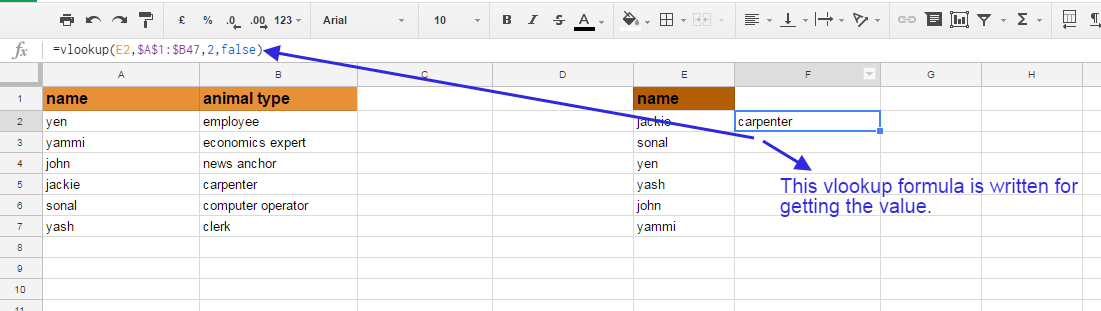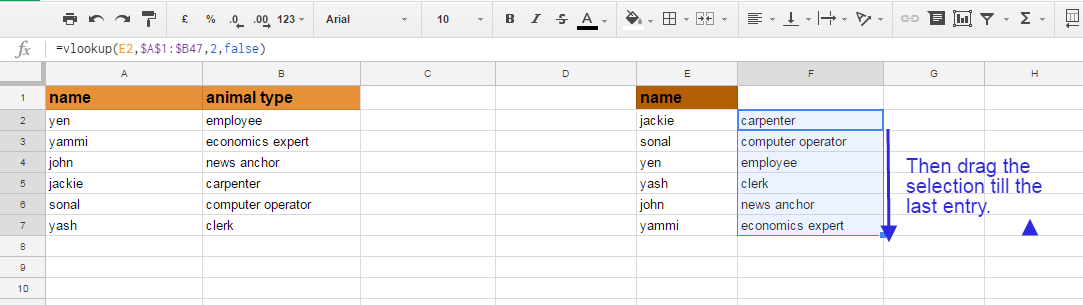### Why do we use the \$ sign before column name and number while writing excel formulas or functions?

When we write \$ before any column name then the reference of that column is locked or the column becomes absolute.

For example when you use SUM(A1, A2) and show the result in A3, now if you copy the formula from A3 and keep it at C3 then the corresponding column will also be changed and they will be SUM(C1, C2) instead of SUM(A1, A2).

So, if you use the \$ sign then you can show the result anywhere regardless to change the position of the input column.

8. IF STATEMENTS

Formula: =IF(logical_statement, return this if logical statement is true, return this if logical statement is false)

OR, =IF(A1>B1, “goal is achieved”, “goal is not achieved”)

These excel formulas are similar to the programming conditional logic. Here we compare a condition if it is true then the statement is correct else it is false.

We can set our goal on the basis of the condition.

See the image below: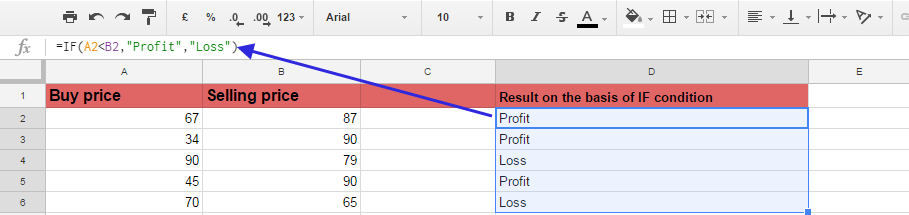9. SUM IF, COUNT IF AVERAGE IF

SUM IF

Formula: =SUMIF(range, criteria, sum_range)  OR  =SUMIF(A2:A7, D2, C2:C7)

This formula is used for finding the result in a cell range on the basis of criteria and summing the cell value matched with the criteria.

See the image below: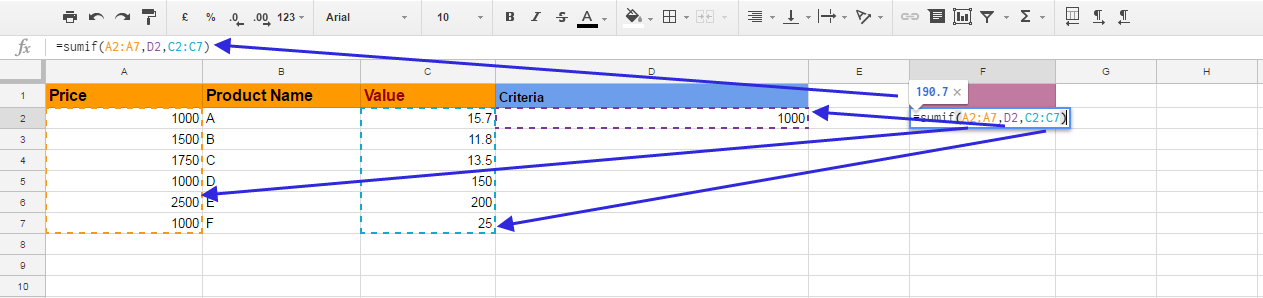COUNTIF

Formula: =COUNTIF(range, criteria)   OR   =COUNTIF(A2;A7, “>1000”)

This formula is used for getting the result in a given cell range with one specific criterion.

See the image below: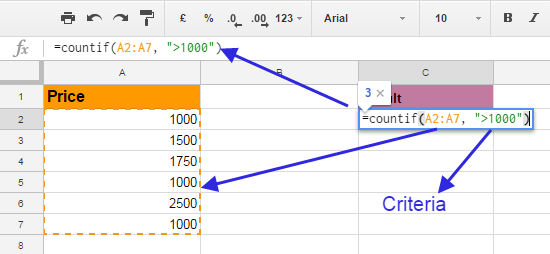AVERAGE IF

Formula: =AVERAGEIF(range, criteria, average_range)   OR   =AVERAGEIF(A2:A7, D2, C2:C7)

See the image below: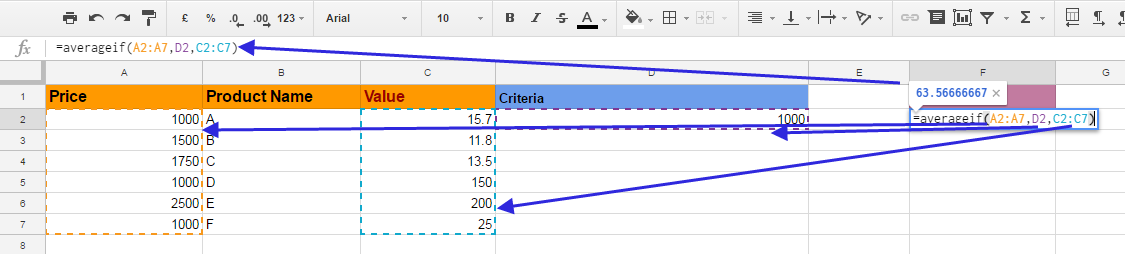10. CONCATENATE

Formula: =CONCATINATE(A1,”-“,B1)

This formula is used for concatenating two strings together with or without any joint like a hyphen sign, underscore and etc.

See the image below: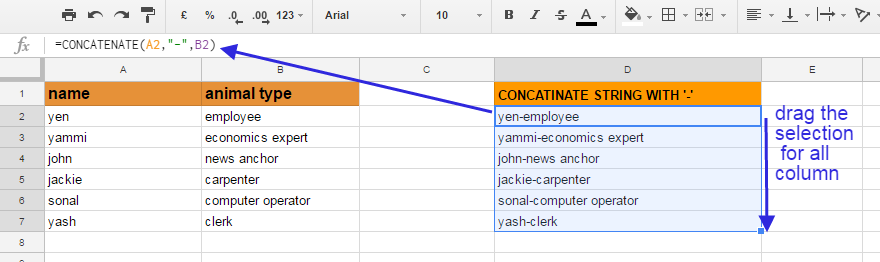So these were the ten basic excel formulas that can enhance your working speed with excel.

You can become an expert in excel, by first, learning the basics so that you could easily grab its more enhanced features.

We will update you with some more features and excel formulas of excel in our upcoming blog post.

## Conclusion:

So in this blog post(Make your job easy with the most useful excel formulas 2023), we have learned about a few important and popular Excel formulas which are often used in our daily routine work while working for our official purpose or study purpose. Excel is a vast and very useful software for storing and manipulating data. With the help of excel formulas, you can make a quick calculation over bulk data, rows, and columns. We have explained 10 very frequently useful excel formulas here in this blog post like sum, Len, count, count-A, Vlookup, If statement and etc.

So finally if we conclude this blog(Make your job easy with the most useful excel formulas 2023) then we have learned how you calculate in excel…?..how you write formulas in excel…? and what are the most used formulas in excel…?

In case of any queries, you can write to us at a5theorys@gmail.com we will get back to you ASAP.

Hope! you would have enjoyed this post ‘Make your job easy with the most useful excel formulas 2023

Please feel free to give your important feedback in the comment section below.

Have a great time! Sayonara!#### Anurag

I am a blogger by passion, a software engineer by profession, a singer by consideration and rest of things that I do is for my destination.